# Eq1

Solve equation: 4(a-3)=3(2a-5)

a =  1.5

### Step-by-step explanation:

4(a-3)=3(2a-5)

4•(a-3)=3•(2•a-5)

2a = 3

a = 3/2 = 1.5

Our simple equation calculator calculates it.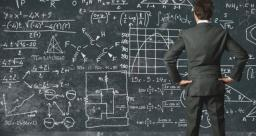Did you find an error or inaccuracy? Feel free to write us. Thank you!

Showing 1 comment:Math studentTips to related online calculators
Do you have a linear equation or system of equations and looking for its solution? Or do you have a quadratic equation?

## Related math problems and questions:

• Equation with one variableSolve the following equation with one unknown: 5(7s + 5) =130
• Equation algebraogram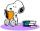Solve the equation: oco + ivo = cita How much has the task of solutions?Why does 1 3/4 + 2 9/10 equal 4.65? How do you solve this?
• If-then equationIf 5x - 17 = -x + 7, then x =
• Do you solve this?Determine area S of rectangle and length of its sides if its perimeter is 102 cm.
• Find xSolve: if 2(x-1)=14, then x= (solve an equation with one unknown)
• Birds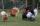On the farm they have a total of 110 birds. Geese and turkeys together is 47. Hens is three times more than the turkey. How much is poultry by species?
• Denominator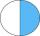Calculate the missing denominator x: (264)/(96) = (11)/(x)Add marks (+, -, *, /, brackets) to fullfill equations 1 3 6 5 = 10 This is for the 4th grade of the primary school - with no negative numbers yet
• Clock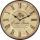What time is now if the time elapsed afternoon is 2/10 of time that elapses before midnight?
• DivideDivide substance 110 cm long to two parts so that it first part is 10 cm longer than the second part and one part will be 10 times longer than the second portion. How long the parts will be?
• Unknown number xyzFind the number that its triple is 24. Solve by equation.
• Perimeter to area 2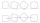Find the area of a square whose perimeter is 100 cm with solve.
• Buns and toastsTwo buns weigh 10 grams more than two toasts. One Bun and two Toast weigh a total of 110 grams. How many grams weigh three toasts? How many grams does a Bun weigh?
• FractionsSort fractions z1 = (6)/(11); z2 = (10)/(21); z3 = (19)/(22) by its size. Result write as three serial numbers 1,2,3.
• Six te 2If 3t-7=5t, then 6t=
• GardensThe garden has the square shape with circumference 124 m. Divide it into two rectangular gardens, one should circumference 10 meters more than the second. What size will have a gardens?